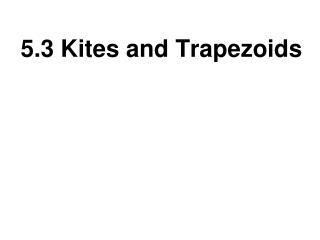DownloadDownload Presentation5.3 Kites and Trapezoids

# 5.3 Kites and Trapezoids

Download Presentation## 5.3 Kites and Trapezoids

- - - - - - - - - - - - - - - - - - - - - - - - - - - E N D - - - - - - - - - - - - - - - - - - - - - - - - - - -
##### Presentation Transcript

1. 5.3 Kites and Trapezoids

2. Kite Investigation Recall the shape of a toy kite. What definition would you write to describe the shape in geometric terms?

3. Kite Investigation Kite Definition • A kite is a quadrilateral that has two pairs of consecutive congruent sides, but the opposite sides are not congruent

4. Vertex Angles Vertex Angles are angles between congruent sides

5. Kite Investigation • Get your supplies • Straight Edge • Patty Paper

6. Kite Investigation • On a piece of patty paper, draw two line segments of different length

7. Kite Investigation • Fold the paper, so that the endpoints are exactly on the fold

8. Kite Investigation • Trace the first two segments through the patty paper

9. Kite Investigation • Unfold the paper and label your kite G,L,A,D L G A D

10. Kite Investigation • Measure each of the four angles • What conclusions can you draw? L G A D

11. Kite Angles Conjecture • The non-vertex angles of a kite are congruent

12. Kite Investigation • Add the diagonals into your diagram • Label the intersecting point M L G M A D

13. Kite Investigation • Measure the angles at point M • What can you conclude about the diagonals? L G M A D

14. Kite Diagonals Conjecture • The diagonals of a kite are perpendicular

15. Kite Investigation • Measure LM and MD • What can you conclude? L G M A D

16. Kite Diagonal Bisector Conjecture • The diagonal connecting the vertex angles of a kite is the perpendicular bisector of the other diagonal

17. Kite Investigation • Fold along the diagonals • Does either diagonal bisect any angles? L G M A D

18. Kite Angle Bisector Conjecture • The vertex angles of a kite are bisected by a diagonal

19. a = 129.5° h= 129.5°

20. a = 118° t= 43°

21. Trapezoid Definition Trapezoid Definition • A trapezoid is a quadrilateral with exactly one pair of parallel sides

22. Trapezoid Vocabulary Trapezoid Definitions • The parallel sides are called bases • A pair of angles that share a base are called base angles A&B, C&D A B C D

23. Trapezoid Consecutive Angles Conjecture • The consecutive angles between the bases of a trapezoid are supplementary

24. Trapezoid Investigation Isosceles Trapezoid • An isosceles trapezoid has congruent legs A B C D

25. Trapezoid Investigation • Get your supplies • Protractor • Straight Edge • Paper

26. Trapezoid Investigation • Draw two parallel lines – at least two inches apart • Use a compass to create two equal length legs • Label the vertices H,O,W,E H O W E

27. Trapezoid Investigation • Measure each pair of base angles angle H = ? angle O = ? angle W = ? angle E = ? H O W E

28. Trapezoid Investigation • What can you conclude about the base angles in an isosceles trapezoid? H O W E

29. Isosceles Trapezoid Conjecture The base angles of an isosceles trapezoid are congruent

30. Trapezoid Investigation • Add the diagonals of the isosceles trapezoid H O W E

31. Trapezoid Investigation • Compare the lengths of the two diagonals • What conclusion can we make? H O W E

32. Isosceles Trapezoid Diagonals Conjecture • The diagonals of an isosceles trapezoid are congruent

33. 53° 127° 127°

34. DF=10 • Angle C=85° • Angle D=95° • Angle F=85°

35. 90° 48°